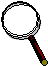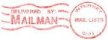# Essential McXtrace Commands

This is a list of the main commands/binaries that the McXtrace install on your system

```mxgui
```
starts the McXtrace GUI
```mcxtrace a.instr
```
interpret the instrument file `a.instr` and output a complete c-program in the file a.c
```mxrun a.instr
```
This utility script does a number of things:
1. Check if the file a.out does not exist or is older than a.instr.
If this is the case:
1.1 Run mcxtrace on a.instr to produce a.c
1.2 Compile a.c to a.out using the c-compiler of choice
2. Run the simulation using 10^6 virtual photons, and prompts the user for input values
```mxrun a.instr par1=12
```
Similar but sets the value of input parameter par1 to 12 and uses the default values for other input parameter. I.e. it does not prompt the user for any more input parameters.
```mxplot
```
Show a dynamic overview plot of the monitors in the working directory
```mxplot dir
```
Show overview plot of data in directory dir
```mxdisplay a.instr
```
Similar to mxrun but do visual tracing of 10^6 photons (a very high number) using the default plotting backend (usually pgplot).
```mxdisplay --format=MATLAB a.instr
```
Do visual tracing using the Matlab backend (if present)